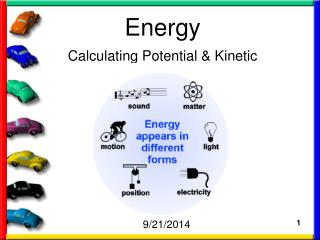DownloadDownload PresentationEnergy

# Energy

Télécharger la présentation## Energy

- - - - - - - - - - - - - - - - - - - - - - - - - - - E N D - - - - - - - - - - - - - - - - - - - - - - - - - - -
##### Presentation Transcript

1. Energy Calculating Potential & Kinetic 9/21/2014 1

2. Today’s Warmup • Potential and Kinetic Energy… a little song and video… • Potential energy, kinetic energy, Rube Goldberg, and OK-Go!

3. Today’s Objectives • To discuss how to calculate potential and kinetic energy- formulas used • Review homework page and complete text assignment

4. Potential and Kinetic Energy Formulas: Notes Notes Notes Notes Notes Notes Notes Notes Notes Notes Summary of the notes and information learned

5. Energy • For there to be movement there must be an input of energy. • Energy is: the ability to cause change. • Energy can light a room, heat a pot of water, move a bicycle…

6. Energy • Energy can classified into two main categories: • Kinetic- the energy of motion • Potential- the stored energy due to position • The physics of roller coaster Cosmeo video…

7. Energy • Potential energy- the stored energy due to position (motion waiting to happen)

8. Potential Energy Work = force X distance How much work is done if we push a heavy box with 21 Newtons of force and move it 12 meters? 252 joules of work

9. Potential Energy

10. Potential Energy

11. Energy • Kinetic energy- the energy of motion

12. Energy • Kinetic energy- the amount of kinetic energy an object has is based on its speed and mass… larger, faster objects have more kinetic energy

13. Kinetic Energy

14. Kinetic Energy The kinetic energy increases as the square of the speed. This means if you go twice as fast, your energy increases by four times. If your speed is three times higher, your energy is nine times bigger. More energy means more force is needed to stop, which is why driving fast is so dangerous. Going 60 mph, a car has four times as much kinetic energy as it does at 30 mph. At a speed of 90 mph you have nine times as much energy as you did at 30 mph.

15. Potential & Kinetic Energy Formulas: Notes Notes Notes Notes Notes Notes Notes Notes Notes Notes Summary of the notes and information learned

16. Today’s Assignment • Complete all lab-work • Complete Potential/Kinetic homework sheet

17. Make it a great day Mustangs!